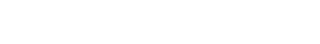## How much electricity does a laptop use per hour in the UK?

A laptop typically uses about 50 watts of electricity, the equivalent of 0.05 kWh. This means that if a laptop is on for eight hours a day, it will cost 5p a day to run the laptop (based on an average energy unit cost of 12.5 p/kWh).

## How much electricity does a PC use in the UK?

A desktop PC typically uses around 100 watts of electricity, the equivalent of 0.1 kWh. This means that if a PC is on for eight hours a day, it will cost 10p a day to run the laptop (based on an average energy unit cost of 12.5 p/kWh).

## How much electricity does a TV use per hour?

170 watts = 0.17 kWh, on for 8 hours a day = 17p a day

## How much electricity do other common appliances use?

Energy is an inescapable expense for all businesses. But have you ever wondered how much each appliance is costing you? Saving electricity (and money) is always easier when you know how much you’re actually using. Although your appliances’ actual energy use depends on make and model (along with other factors like temperature conditions and the age of the appliance), these figures will give you an idea of how much it costs to run the appliances in your office.

## How many watts do common appliances use?

 Appliance Watts Immersion heater 3000W Oil-filled radiator 1500-2500W Dishwasher 1050-1500W Vacuum cleaner 500-1200W Toaster 800-1500W Kettle 2200-3000W Microwave 600-1500W Oven 2000-2200W Dehumidifier 300-700W Extractor fan 5-36W Freezer 150W Laptop 20-50W Desktop computer 80-150W Tablet (charge) 10W Broadband router 7-10W Smartphone (charge) 2.5-5W

What is a watt?

## How to calculate the amount of energy used by each appliance

Power rating in kW x hours used per day = kWh

## How to calculate the energy cost of each appliance

kWh per day x unit cost = electricity cost per day

## For example…

Take a 150-watt fridge and an energy unit cost of 12.5 p/kWh. The fridge runs 24 hours per day.

150W = 0.15kW
0.15 kW x 24 hours = 3.6 kWh
3.6 kWh x 12.5p = 45p per day

## How Much Energy Do My Business Appliances Use?

Common appliances and their typical power ratings and cost (based on an average unit cost of 12.5p/kWh.)

### How much energy does a server use?

1000 watts = 1kWh, on for 24 hours per day = £3 per day = £1095 per year

### How much electricity does a desktop PC use?

100 watts = 0.1 kWh, on for 8 hours per day = 10p per day

### How much electricity does a laptop use?

50 watts = 0.05 kWh, on for 8 hours per day = 5p per day

### How much does it cost to use a microwave oven?

1 kWh per use = 12.5p for every ready meal

### How much electricity does it take to boil a kettle?

0.11 kWh to heat 1 litre of water = 1.4p for 4 cuppas

### How much does it cost to run an air conditioner?

4000 watts = 4kWh, on for 8 hours a day =£4 per day

### How much electricity does a lightbulb use?

60 watt = 0.06kWh, on for 8 hours per day = 6p per day

## Which appliances use more electricity?

As a general rule, appliances which produce heat or have moving parts use more electricity than those that produce sound or light.

## What is a power rating?

Every electrical appliance has a power rating which tells you the amount of electricity it needs to work. The power rating is generally given in watts (W) or kilowatts (kW). There are 1000 watts in one kilowatt. The amount of electricity each appliance uses is measured in time – kilowatt hours (kWh). UK energy suppliers measure energy consumption by kWh and charge a unit cost per kWh.

## How many watts do my appliances use?

See the infographic below to find out…